# How to use Loops in Simulink MATLAB : tutorial 7

In this tutorial, I will explain you the working of conditional loops and how to implement them using Simulink. At the beginning you are provided with a brief introduction of loops, and where we can use them also a basic introduction of loops in other programming languages and their working principles. After that loops are implemented on Simulink’s platform and their working is explained step by step. After simple implementation loops are implemented using examples of counter. A counters named as up counter is implemented on Simulink and its outputs is displayed. At the end I gave a simple exercise to perform it on your own, this will help you strengthen your concepts regarding algebraic loops in Simulink.

Introduction to Algebraic loops

Algebraic loops mean to perform a certain task iteratively up to a certain number of times or un till a specific condition is fulfilled. In Simulink there is no proper block to implement loops, however we can design our own loop in which the output is fed to the input with the delay in between to remove any ambiguity. In order to make the loop error free we must provide a condition which will decide whether the loop will be continued or stopped.

In other programming languages the loops are named as for loop and while loop and at this stage of programming I assume that you are familiar with these loops.

Explanation with a program:

### Designing an up counter with Simulink

Lets’ now move towards the programing part. I will now perform here a simple example which will help you understand the working of loops in simulink. Open MATLAB and then open Simulink. In Simulink click on the library browser icon and open library browser as we have been doing in previous tutorials. From the library browser click on the commonly used blocks section as we have been doing in previous tutorials and then select the constant block from that section as shown in the figure below,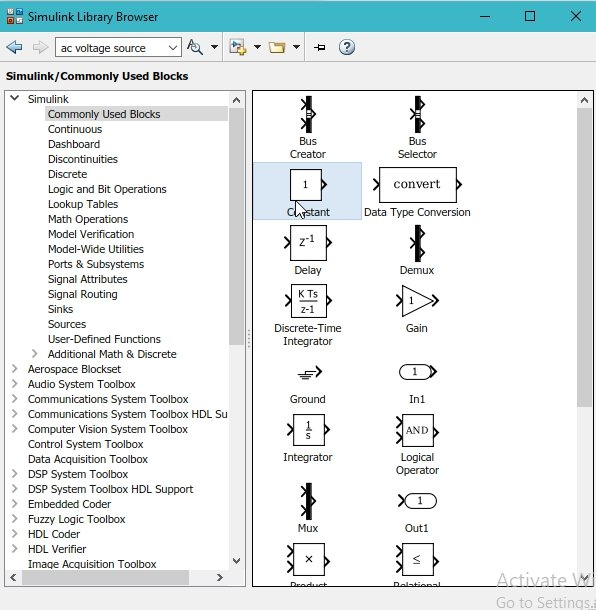Figure 1: Constant block

• This block will be used as the size of the step of the counter i.e. If o set it to 2 the counter will change its value in steps of 2 as you will see shortly, once the model of the up counter is complete. In order to design an algebraic loop for the implementation of the up counter we need an add block which will add 1 to the previous value after each iteration of the loop. From the library browser select the math operation section as shown in the figure below,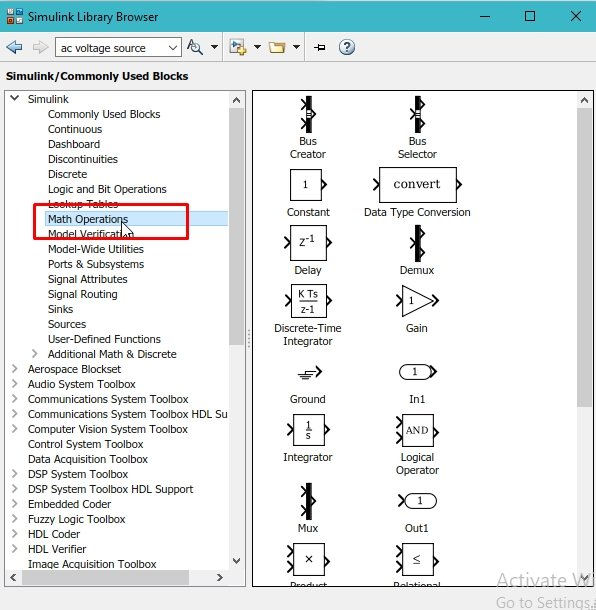Figure 2: Math operation

• This add block will add up the previous value of the counter to the constant block placed previously to complete one iteration. Now if we directly attach the output of the add block to its input Simulink will consider it as an error because both the operations are implemented on the same instant. Therefore, we need a delay block that will add a delay in the addition of the previous value. From the library browser select the commonly used blocks section and from that section select the delay block as shown in the figure below,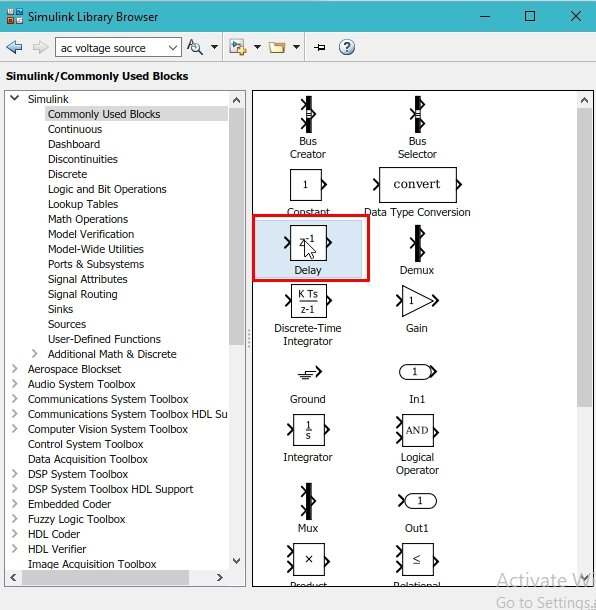Figure 4: Delay block

• This will add the selected block to the current model. Now in order to see the output of the counter we also need some output display. This display block, however, will only show the most recent output after the execution that’s why it is not suitable here. We will use the scope block of Simulink to see all the process of the loop in one window. Therefore, from the commonly used blocks section select the scope block as shown in the figure below,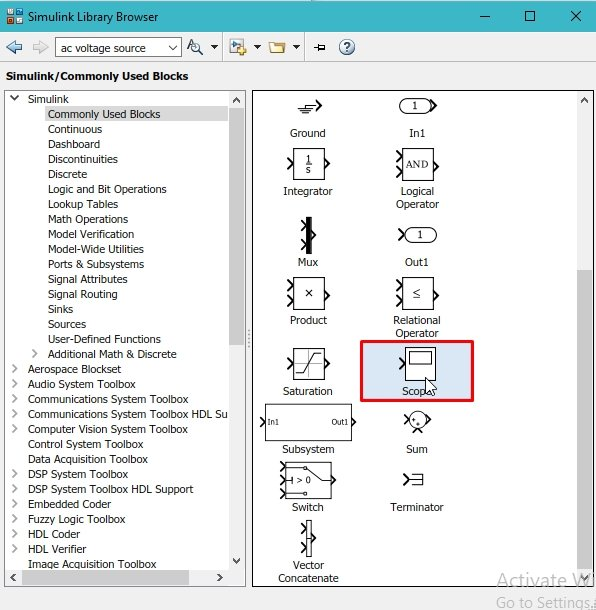Figure 6: Scope block

Figure 7: Placed components

Figure 8: Flipping block

Figure 9: Block diagram

Figure 10: Output

• The problem with the counter is that there is no resetting condition of this counter. A counter with no resetting condition is of no use. It will start updating the previous value to infinity. We must need to provide it some limiting value after which it must reset its value to zero and start over again. For that purpose, we need a conditional statement as we have used in previous tutorial. From the library browser select the signal routing section as shown in the figure below,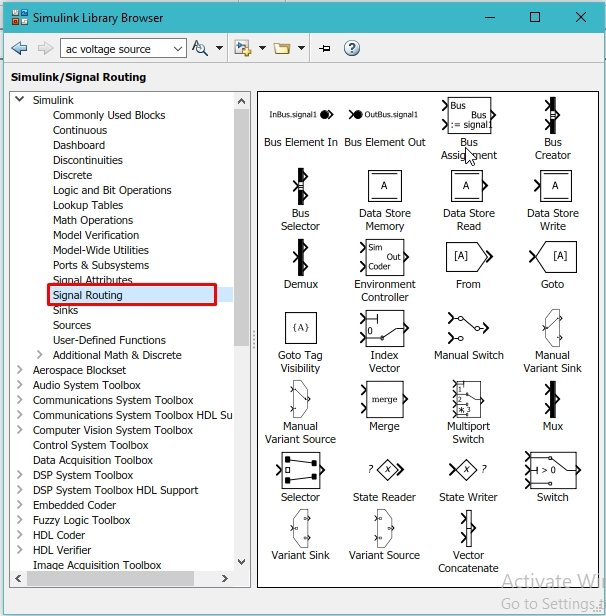Figure 11: Signal routing

Figure 12: Switch block

Figure 13: Threshold value

Figure 14: False condition

Figure 15: True condition

Figure 16: Conditional up counter

Figure 17: Output of up counter

This is a simple up counter which will start from zero and end to the value provided by us in the switch block.

Exercise:

• Design an down counter using MATLAB’s Simulink.

(Hint: You might need to change the condition of the switch block from > to <)